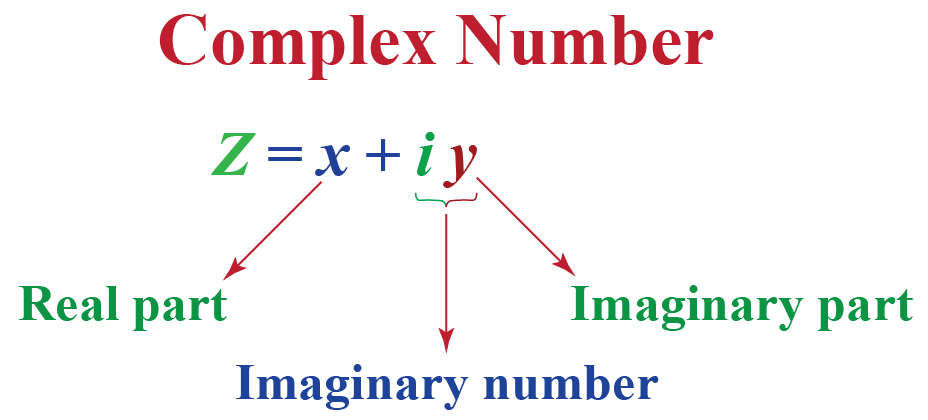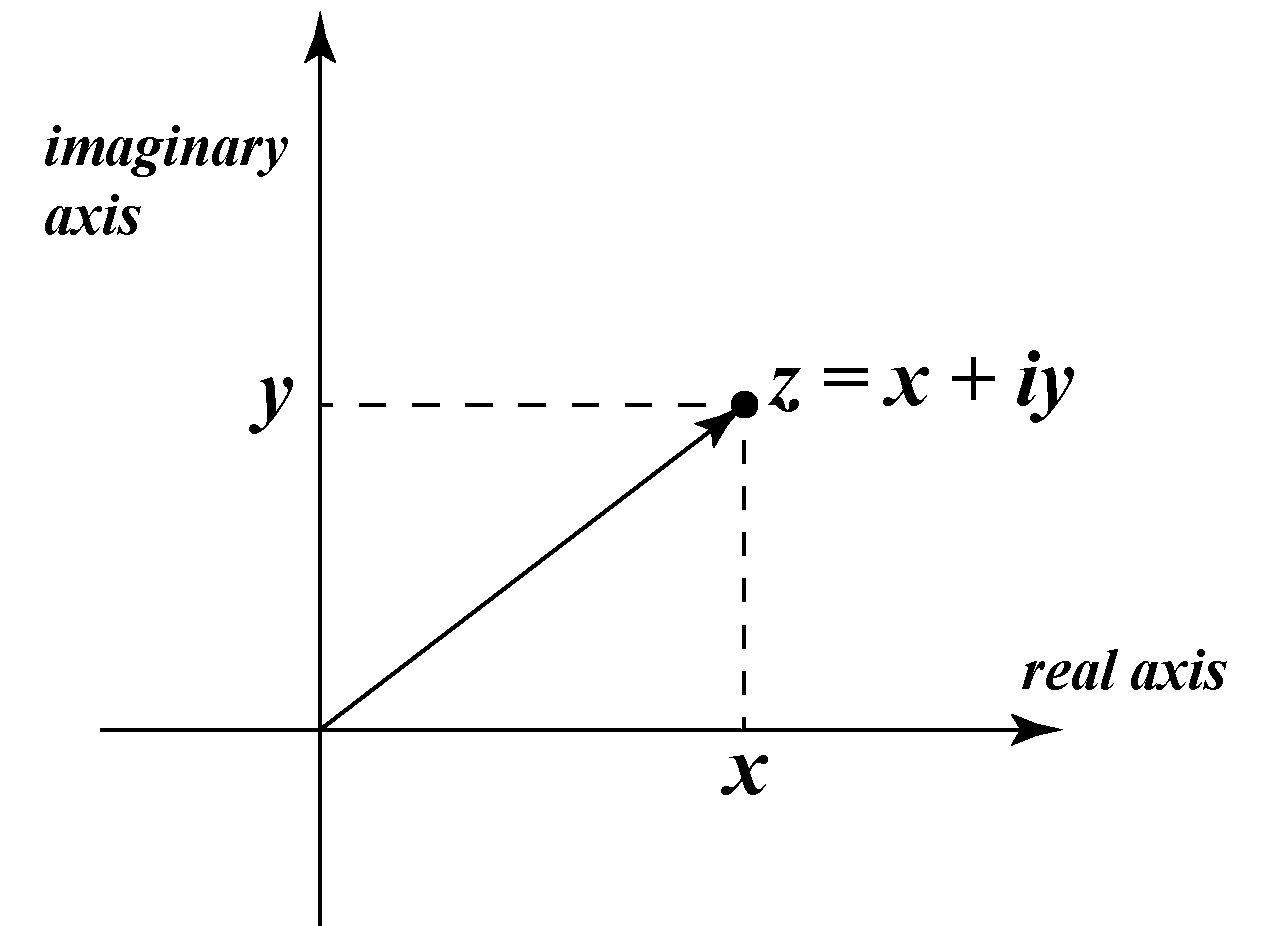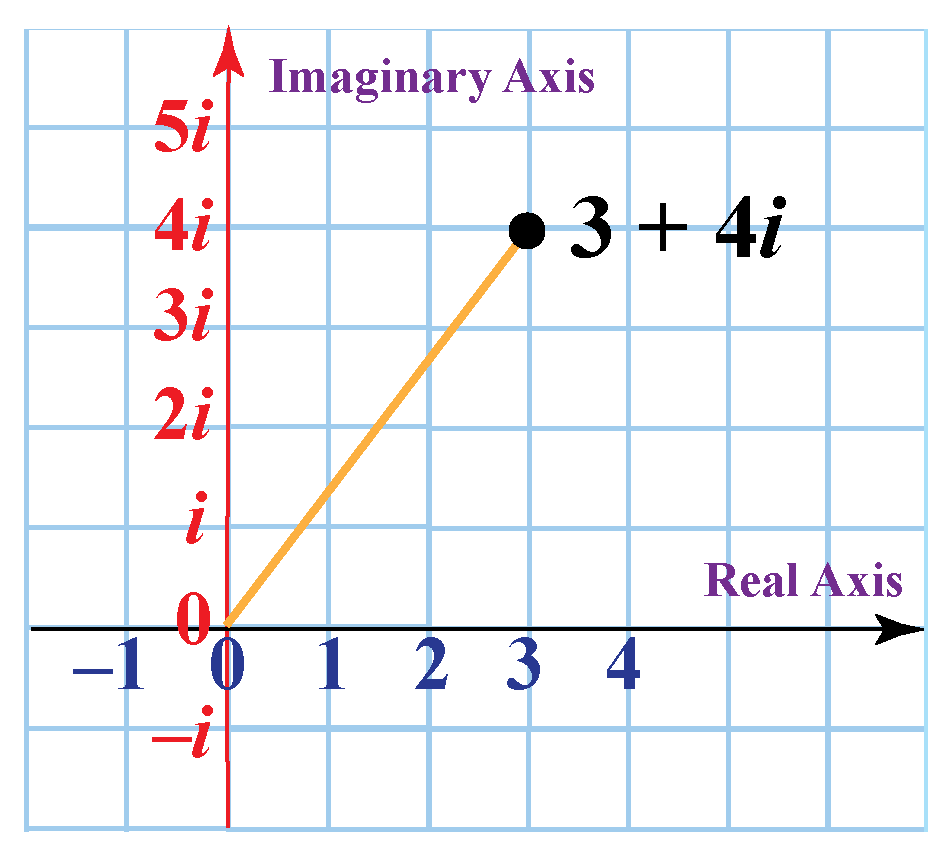# Graphing Complex Numbers

Graphing Complex Numbers

A complex number is the sum of a real number and an imaginary number; that is, a complex number is of the form $$x+iy$$ and is usually represented by $$z$$.

Here, both $$x$$ and $$y$$ are real numbers.

• $$x$$ is called the real part.
• $$y$$ is called the imaginary part.
• $$iy$$ is an imaginary number.In this mini-lesson, we will explore the world of graphing complex numbers on a complex plane. We will learn plotting complex numbers on a complex plane, complex plane grapher, graphing imaginary numbers, and discover other interesting aspects of it.

You can check out the interactive simulations to know more about the lesson and try your hand at solving a few interesting practice questions at the end of the page.

## Lesson Plan

 1 What Is a Complex Plane? 2 Important Notes on Graphing Complex Numbers 3 Solved Examples on Graphing Complex Numbers 4 Thinking out of the Box! 5 Interactive Questions on Graphing Complex Numbers

## What Is a Complex Plane?

Every complex number can be represented by a point in the XY-plane.

The complex number $$x+iy$$ indicates the point $$(x,y)$$ in the XY-plane.The plane where a complex number is assigned to each of its points is called a complex plane.

A complex plane is also called an argand plane.

## How to Plot Complex Numbers as Points on a Complex Plane?

### Graphing Complex Numbers on a Complex Plane Grapher

In this section, you will learn plotting complex numbers on a complex plane.

### Steps to Plot Complex Numbers

Follow the steps mentioned below to plot complex numbers on the complex plane.

1. Determine the real part and imaginary part of the given complex number. For example, for $$z=x+iy$$, the real part is $$x$$ and the imaginary part is $$y$$.

2. Form an ordered pair where the first element is the real part and the second element is the imaginary part. For example, for $$z=x+iy$$, the ordered pair is $$(x,y)$$

3. Plot the point $$(x,y)$$ on the plane.

### Examples

Let us consider the complex number $$z=3+4i$$.

The real part is 3 and the imaginary part is 4.

So, the ordered pair is (3, 4).

The complex number $$z=3+4i$$ is represented in the graph below.Complex Numbers
Complex Numbers
Complex Numbers
grade 10 | Questions Set 2
Complex Numbers
grade 10 | Questions Set 1
More Important Topics
Numbers
Algebra
Geometry
Measurement
Money
Data
Trigonometry
Calculus
More Important Topics
Numbers
Algebra
Geometry
Measurement
Money
Data
Trigonometry
Calculus
Learn from the best math teachers and top your exams

• Live one on one classroom and doubt clearing
• Practice worksheets in and after class for conceptual clarity
• Personalized curriculum to keep up with school